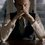# Wau : The most amazing, ancient and singular number; and more

I am sharing a video from ViHart which is very good and a must for an exploring mathematician.

And yes, the bonus one is the following link:

https://en.wikipedia.org/wiki/Listofunsolvedproblemsin_mathematics

I believe that one and especially, the Brilliant Mathematicians here should have a look and at least try to solve them and become the next generation Andrew Wiles.Note by Kartik Sharma
7 years, 1 month ago

This discussion board is a place to discuss our Daily Challenges and the math and science related to those challenges. Explanations are more than just a solution — they should explain the steps and thinking strategies that you used to obtain the solution. Comments should further the discussion of math and science.

When posting on Brilliant:

• Use the emojis to react to an explanation, whether you're congratulating a job well done , or just really confused .
• Ask specific questions about the challenge or the steps in somebody's explanation. Well-posed questions can add a lot to the discussion, but posting "I don't understand!" doesn't help anyone.
• Try to contribute something new to the discussion, whether it is an extension, generalization or other idea related to the challenge.

MarkdownAppears as
*italics* or _italics_ italics
**bold** or __bold__ bold
- bulleted- list
• bulleted
• list
1. numbered2. list
1. numbered
2. list
Note: you must add a full line of space before and after lists for them to show up correctly
paragraph 1paragraph 2

paragraph 1

paragraph 2

[example link](https://brilliant.org)example link
> This is a quote
This is a quote
    # I indented these lines
# 4 spaces, and now they show
# up as a code block.

print "hello world"
# I indented these lines
# 4 spaces, and now they show
# up as a code block.

print "hello world"
MathAppears as
Remember to wrap math in $$ ... $$ or $ ... $ to ensure proper formatting.
2 \times 3 $2 \times 3$
2^{34} $2^{34}$
a_{i-1} $a_{i-1}$
\frac{2}{3} $\frac{2}{3}$
\sqrt{2} $\sqrt{2}$
\sum_{i=1}^3 $\sum_{i=1}^3$
\sin \theta $\sin \theta$
\boxed{123} $\boxed{123}$

Sort by:

Yeah, what's really amazing is that it even plays a role in Einstein's General Relativity! Here's the equation
$F=\dfrac { 8\pi T }{ G }$ where $F$ is this amazing Wau number, $G$ is the curved spacetime Einstein tensor, and $T$ is the geometrized stress-energy tensor! Will wonders of this strange number ever cease!

- 7 years, 1 month ago

what is this? I didn't know anything about this equation. What is G and T? I cannot understand that still. By the way, how we approached to this number, just how? I never understand this.

- 7 years, 1 month ago

Kartik, I hate to disappoint you, but "Wau", or $F$, is just $1$. In the video, you can see where it gives it away $F={ e }^{ 2\pi i }=1$ So, Einstein's equation is really $G=8\pi T$, which is the basis of his General Relativity. That is, curved spacetime and gravity are one and the same. I was just having fun with this. And so was the person or people responsible for this video. Wau is nothing anything more special than the number $1$.

- 7 years, 1 month ago

He's already explained what the variables stand for. Tell me, if there is an infinte decimal approximation of Wau please?

- 7 years, 1 month ago

I don't know and that's what I supposed to ask him. BTW, I actually asked what does "curved spacetime Einstein tensor" and "geometrized stress-energy tensor" mean?

BTW, you haven't told me what I asked you - the 2 questions.

- 7 years, 1 month ago

Wau is $1$. If that is all you care about you can do something else. But for those who want to know more here's a link to something explaining it. https://www.youtube.com/watch?v=-eS8-1A47Z0. Or you can try proving it from the examples in the video. Which is https://www.youtube.com/watch?v=GFLkou8NvJo

- 3 years, 7 months ago